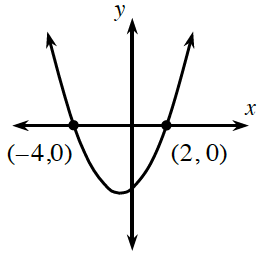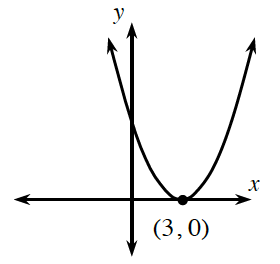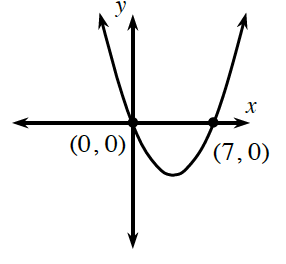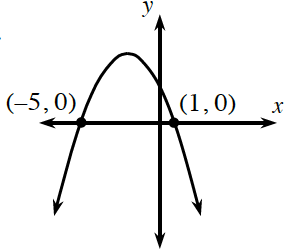### Home > INT2 > Chapter 5 > Lesson 5.1.5 > Problem5-55

5-55.

QUALITY CONTROL, Part Three

Lots O’Dough, a wealthy customer, would like to order a variety of parabolas. However, he is feeling pressed for time and says that he will pay you lots of extra money if you complete his order for him. Of course you agree! He sends you sketches of each parabola that he would like to receive. Determine a possible equation for each parabola so that you can pass this information on to the Function Factory.

1.The $x$-intercepts are a good place to find factors of the quadratic equation.

$y = (x + 4)(x - 2) = x^2 + 2x - 8$

1.There is only one $x$-intercept, but this is a parabola, so the equation needs to be quadratic.

$y = (x − 3)(x - 3)$

1.Use the $x$-intercepts to help you write a possible equation.

1.Notice that this parabola opens downward.